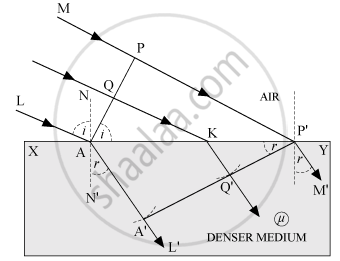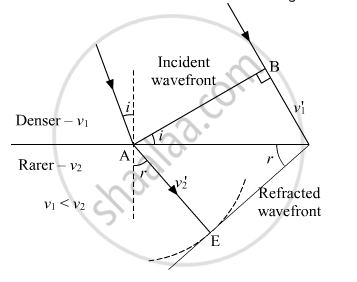# Define the Term, "Refractive Index" of a Medium. Verify Snell'S Law of Refraction When a Plane Wavefront is Propagating from a Denser to a Rarer Medium. - Physics

Define the term, "refractive index" of a medium. Verify Snell's law of refraction when a plane wavefront is propagating from a denser to a rarer medium.

#### Solution

Refractive index of a medium may be defined as the ratio of the speed of light in air and the speed of light in the given medium. It doesn’t have a unit.

Refraction On The Basis Of Wave TheoryConsider any point Q on the incident wavefront.

Suppose when disturbance from point P on incident wavefront reaches point P' on the refracted wavefront, the disturbance from point Q reaches Q' on the refracting surface XY.

Since P'A' represents the refracted wavefront, the time taken by light to travel from a point on incident wavefront to the corresponding point on the refracted wavefront should always be the same. Now, the time taken by light to go from Q to Q' will be

t = "QK"/c + "KQ'"/"v"      ....(i)
In right-angled ΔAQK,
∠QAK = i
QK = AK sin i          … (ii)

In right-angled ΔP'Q'K,
∠Q'P'K = r
KQ' = KP' sin r          ...(iii)

Substituting (ii) and (iii) in equation (i),

t = ("AK"sin i)/(c) + ("KP"'sin r)/("v")

Or, t = ("AK"sin i)/(c) + (("AP'"- "AK")sin r)/v  ....(∵ "KP"' = "AP"' - "AK")

Or, t = ("AP"')/(c) sin r + "AK" (sin i/c - sin r /"v")    ....(iv)

The rays from different points on the incident wavefront will take the same time to reach the corresponding points on the refracted wavefront i.e., t given by equation (iv) is independent of AK. It will happen so, if

sin"i"/c - sin"r"/"v" = 0

sin "i"/sin "r" = c / "v"

However, c/"v" = mu

mu = sin"i"/sin"r"

This is the Snell’s law for refraction of light.Concept: Snell’s Law
Is there an error in this question or solution?